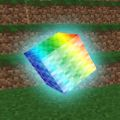# The Physics of Minecraft: Gravity

• 15,150 views, 1 today
• 4
• 2
• 5
Hello good folk, Professor Sparkle here. As you might have noticed from the title of the thread, I study physics. Physics is the study of the natural world using mathematics. Since physics applies to almost all aspects of the physical world, Minecraft caused me to raise a question.

Minecraft and Physics: Will it blend? That is the question.

And yes, it blends!

Gravity. It's the thing that makes your grandmother's apple pie fall off the table and onto the ground, resulting in you receiving a severe beating from said grandmother. Unsurprisingly, this unforgiving force exists in Minecraft as well. But to what extent?

Scientifically scientific experiments (of science, no doubt) were carried out to analyze the force due to gravity in the Minecraft universe. These experiments were documented in the video above, and will not be reiterated for the sake of brevity. Time is money, children!

First, let us present the primary equation for a projectile:This equation states that the time a projectile (in our case, the feeble sand particle) remains in the air is dependent on the distance traveled, the acceleration due to gravity, and any initial velocities and displacements. Since there are no initial velocities or displacements, because we are beginning our reference from the highest point of the sand particle's projectile path, we may strike them down. I SAID STRIKE THEM DOWN.

This striking down leaves us with the following equation:Since we wish to solve for a, the acceleration due to gravity, we must now apply my old arch-nemesis Algebra in order to rearrange the equation, giving us the following:Plugging in the data collected in the above experiment, the equation simplifies down to the following:This leads us to the conclusion that the acceleration due to gravity in Minecraft is 18 meters per second squared! This applies in the overworld at least - I do not wish to speculate on the Devil Physics that occurs in the Nether, where not even time is sacred.

But there is so much more that could be explored! Alas, it is time for my nap, so until next time, this has been Professor Sparkle, educating you ruffians Minecrafters one day at a time! Adieu!

[tl;dr my friend and I tested gravity in Minecraft and thought it would be fun to present it]

1
11/07/2019 1:36 amhistory
Level 1 : New Miner
kevweasleyEverything you said is correct, except you can't assume air resistance is equal to zero. If you test your answers you will find that g=18m/s^2 doesn't accurately predict how long you will be falling. In order to accurately predict how long you will fall you need to figure out the mass of the player (m), the acceleration due to gravity (g), and the coefficient of air resistance (k).

My Procedure
There is probably a way with differential calculus to determine m, g and k. But I decided to collect the data, and then regress the points to determine the needed values.
1. To start, I used the command /tellraw @p {"nbt":"FallDistance","entity":"@p"} in a command block, set to an 8-tick clock, to print to the console how long I, the player, was falling. I then teleported my self to a height of 2000 blocks, and let myself free fall.
2. Now that I have around 70 data points for displacement over time, I can regress the equation to find m, g, and k.

Regressing The data
The equation for free fall with air resistance is h=(m/k) * ln(cosh(t/sqrt(m/gk))).
Using Desmos, I regressed all my points and determined that:
• m = 2.105kg
• g = 22.46 m/s^2
• k = 0.011 kg/m

These values fit the curve with an R^2 = 1. If you take the 2nd derivative of this function you will get the acceleration. Because the player has such a small mass, the acceleration is 22.46 m/s^2 at t=0 and it drops to 20.69 m/s^2 at t=1. and at t=1.664 acceleration is 18 m/s^2.

Like, you I was interested, and got similar answers when trying to use simple 1D motion. But I am glad I dug deeper and found out all of this. Hope you found this interesting!
1
01/23/2018 12:38 am
Level 1 : New Miner
YOLOSTEALTHBAWSok so, before even looking at this post, i calculated this the same way but got different results, i decided to check online to see if i was right but then was confused, so i looked into it, i tried the same height as you and got your answer, i had tried from 20 blocks and gotten twice as much, i then tried from 63 blocks and got around 23 m/s^2, I think physics in minecraft is broken or just doesn't follow our universe' laws of gravitation
1
11/02/2014 12:42 pm
Level 16 : Journeyman Engineer
567legodudeCould you figure out what the gravity is for projectiles such as arrows?
1
06/27/2012 10:06 pm
Level 28 : Expert Dragonborn
epicdude32I am a kid who loves physics and that was simply brilliant!!!!!!!!!!!!!!!!!!!!!!!!!!!!!!!!!!!!
1
12/31/2011 9:40 pm
Level 22 : Expert Spelunker
ZedeikUhhhhhh..... You lost me at the first equation :)